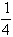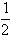Support the Monkey! Tell All your Friends and TeachersHome MonkeyNotes Printable Notes Digital Library Study Guides Study Smart Parents Tips College Planning Test Prep Fun Zone Help / FAQ How to Cite Request a New Title

7.7 Theoretical Distributions

Random variable and probability distributions

Random variables : When we assign a number to each point of a sample space, we have a function which is defined on the sample space. This function is called a random variable (or stochastic variable).

It is usually denoted by bold letters like X or Y.

Consider two independent tosses of a fair coin

\ S = { (HH), (HT), (TH), (TT) }

Let X denote the number of heads.

Then X (HH) = 2, X (HT) = 1, X(TH) = 1 and X(TT) = 0

i.e. X = 0, 1, 2. Here X is known as a random variable. Thus a random variable is one, which denotes the numerical value of an outcome, of a random experiment.

(1) If the random variable X takes only finite values or countably infinite values, then X is known as a discrete variable

(2) If the random variable takes the uncountably infinite values between a specified range or limit, it is called as continuous random variable.
For example : X denotes the age of a person.Your browser does not support the IFRAME tag.

Discrete probability distribution

Return to the above given example of tossing of a fair coin twice.

S = { (HH), (HT), (TH), (TT) }

Then X (HH) = 2, X (HT) = 1, X (TH) = 1 and X(TT) = 0

Also P (HH) =, P (HT) =, P (TH) =and P (HH) =The probability distribution :- Number of Heads

X         P(x)

0 ®or 0.25

1 ®+=or 0.50

2 ®or 0.25

Index

7. 1 Introduction
7.2 Trial
7.3 Sample Space
7. 4 Definition of Probability
7. 5 The Laws of Probability
7. 6 Conditional Probability
7. 7 Theoretical Distribution
7. 8 Binomial Distribution
7. 9 Normal Distribution
Chapter 8Search: All Products Books Popular Music Classical Music Video DVD Toys & Games Electronics Software Tools & Hardware Outdoor Living Kitchen & Housewares Camera & Photo Cell Phones Keywords: